# “The Knowledge Library”

Knowledge for All, without Barriers…

# “The Knowledge Library”

Knowledge for All, without Barriers……….

# DI & Caselet-10

Directions (1 – 5): Study the following paragraph carefully and answer the following questions.

A race is conducted among three boats A, B and C. The race is travelling from x to y and coming back to the same place x. C won the race. After 4 hrs of C’s arrival, B finished the race but the time for B’s downstream and C’s upstream is same. After 7 hrs 30 minutes of B’s arrival, A finished the race but the time for A’s downstream and B’s upstream is same. Total race distance (Downstream + Upstream) is 240km. The speed of the stream is 2km/hr.

1) What is the speed of boat A?

a) 16

b) 12

c) 10

d) 14

e) 20

2) What is the total time taken by B to complete the race?

a) 27 hours 50 minutes

b) 21 hours 50 minutes

c) 21 hours 30 minutes

d) 17 hours 30 minutes

e) 27 hours 30 minutes

3) Find the distance travelled by the boat C in 90 minutes in still water

a) 21 km

b) 28 km

c) 15 km

d) 30 km

e) 27 km

4) Find the ratio of upstream speed of boat A to the downstream speed of boat B

a) 2:3

b) 1:2

c) 5:7

d) 4:7

e) 3:7

5) What is the distance travelled by boat C if it travelled for 5 hours upstream and 4 hours downstream?

a) 160 km

b) 164 km

c) 124 km

d) 128 km

e) 120 km

Data interpretation

Direction (6 – 10): Study the following data carefully and answer the questions:

There are five containers P, Q, R, S and T that contains the mixture of milk and water. Bar graph given below shows the average quantity of milk and water in the containers and it also shows the difference between quantity of milk and water in those containers.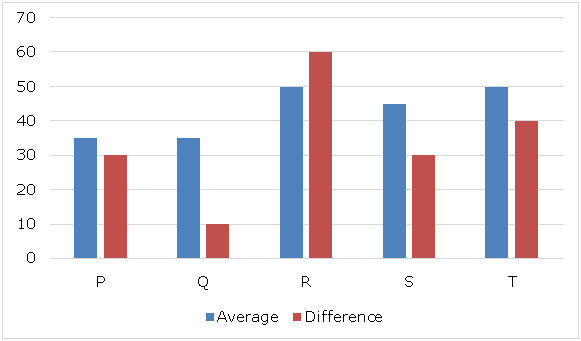Note: All the quantities of milk and water are multiple of ‘5’ and all the values are in litres.

• Quantity of milk is more than quantity of water in every container.

6) If mixtures from containers P and Q are mixed in the ratio 2: 5 to form mixture A and difference between milk and water in the mixture A is 24.2 litres, then what is the total selling price of mixture A when sold at Rs.25 per litres?

a) Rs.3125

b) Rs.2695

c) Rs.2785

d) Rs.4225

e) None of these

7) Mixtures Q and R are mixed in the 28: X to form mixture B and when mixture B is sold at the price of pure milk, then profit percent earned is 58(1/3)%, then what is the value of ‘X’?

a) 15

b) 5

c) 10

d) 20

e) None of these

8) When 20 litres and 10 litres respectively is taken out from mixture R and mixture T and replaced with same amount of water. If this process is repeated one more time, then what will be the difference between amount of pure milk left in mixture R and that in mixture T?

a) 7 litres

b) 6 litres

c) 4.5 litres

d) 5.5 litres

e) None of these

9) If mixture R and S are mixed in the ratio 5: 9 to form mixture C and mixture C is sold at the price equal to 85% of the price of pure milk, then what will be the profit percent earned by the seller after selling the mixture C?

a) 21%

b) 19%

c) 23%

d) 27%

e) None of these

10) Mixtures R and T are mixed in certain ratio to form mixture D. From 280 litres of mixture D, 56 litres are taken out and replaced with same amount of water. This process is repeated one more time and now, quantity of water in the mixture D becomes 134.4 litres, then in what ratio mixture R and T are mixed to form mixture D?

a) 1: 1

b) 3: 2

c) 4: 3

d) 5: 4

e) None of these

Directions (1 – 5):

Let Sa, Sb and Sc be the speed of boat A, boat B and boat C in still water respectively.

By given data, Speed of stream = 2km/hr

C’s upstream = B’s downstream

Sc-2 = Sb+2

Sc – Sb = 4 —– (1)

B’s upstream = A’s downstream

Sb – 2 = Sa + 2

Sb – Sa = 4 —–(2)

Adding the above two, Sc – Sa = 8 ——(3)

Time difference between C and B = 4 hours

Time for B’s upstream – Time for C’s downstream = 4

(120/(Sb-2)) – (120/(Sc+2)) = 4

120[(1/(Sb – 2)) – (1/(Sc + 2))] = 4

(Sc+2 – Sb+2)/((Sb-2)(Sc+2)) = 1/30

(Sc – Sb+4)/(Sb*Sc -2Sc+2Sb-4) = 1/30

(4+4)/(Sb*Sc -2(Sc – Sb) -4) = 1/30

Sb*Sc – (2*4) – 4 = 30*8

Sb*Sc = 252

(Sc + Sb)2 = (Sc – Sb)2 + 4Sc*Sb                         [(a + b)2 = (a – b)2 + 4ab]

= 16 + 4*252

(Sc + Sb)= 1024

Sc + Sb = 32 —- (4)

Adding (1) and (4) Sc = 18

Sb = 14

Sa = 10

Speed of boat A = 10 km/hr

Downstream speed of B = 16km/hr

Upstream speed of B = 12 km/hr

Time = (120/16) + (120/12) = 7.5 + 10 = 17.5hrs

Distance travelled by Boat C in still water in 90 minutes = 18*(90/60) = 27 km

Sc = 18

Sb = 14

Sa = 10

Speed of A upstream = 8km/hr

Speed of B downstream = 16 km/hr

Ratio = 8:16 = 1:2

Sc = 18; Sb = 14; Sa = 10

Upstream speed of C = 16 km/hr

Downstream speed of C = 20 km/hr

Distance travelled = (16*5) + (20*4) = 160km

Directions (6 – 10):

Total quantity of milk and water in container P = 35 * 2 = 70 litres

Amount of milk in container P = (70 + 30)/2 = 50 litres

Amount of water in container P = (70 – 30)/2 = 20 litres

Total quantity of milk and water in container Q = 35 * 2 = 70 litres

Amount of milk in container Q = (70 + 10)/2 = 40 litres

Amount of water in container Q = (70 – 10)/2 = 30 litres

Total quantity of milk and water in container R = 50 * 2 = 100 litres

Amount of milk in container R = (100 + 60)/2 = 80 litres

Amount of water in container R = (100 – 60)/2 = 20 litres

Total quantity of milk and water in container S = 45 * 2 = 90 litres

Amount of milk in container S = (90 + 30)/2 = 60 litres

Amount of water in container S = (90 – 30)/2 = 30 litres

Total quantity of milk and water in container T = 50 * 2 = 100 litres

Amount of milk in container T = (100 + 40)/2 = 70 litres

Amount of water in container T = (100 – 40)/2 = 30 litres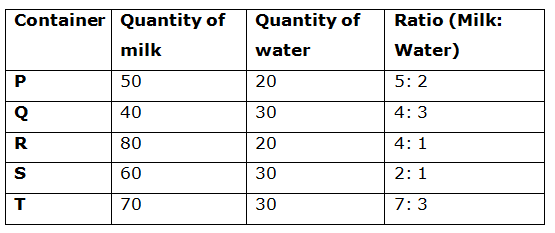Let part of milk in mixture A = A

By the rule of alligation: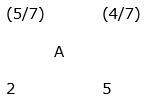[(4/7) – A]: [A – (5/7)] = 2: 5

(20/7) – 5A = 2A – (10/7)

7A = (30/7)

A = 30/49

Ratio of milk to water in mixture A = 30: (49 – 30) = 30: 19

Total quantity of mixture A = 24.2 * [(30 + 19)/(30 – 19)] = 107.8 litres

Total selling price of mixture A = 107.8 * 25 = Rs.2695

Since, profit percent earned after selling mixture B at the cost of pure milk is 58(1/3)%.

Hence, ratio of milk to water in mixture B = 100: 58(1/3) = 100: 58(1/3) = (300/3): (175/3) = 12: 7

By the rule of alligation: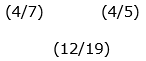Ratio of milk to water = [(4/5) – (12/19)]: [(12/19) – (4/7)]

= [(76 – 60)/95]: [(84 – 76)/133]

= (16/95): (8/133)

= 14: 5

= 28: 10

Hence, X = 10

When 20 litres of mixture is taken out and replaced with same amount of water twice from mixture R, then amount of pure milk left in mixture R:

= 80(1 – 20/100)2

= 51.2 litres

When 10 litres of mixture is taken out and replaced with same amount of water twice from mixture T, then amount of pure milk left in mixture T:

= 70(1 – 10/100)2

= 56.7 litres

Required difference = 56.7 – 51.2 = 5.5 litres

Let part of milk in mixture C = C

By the rule of alligation: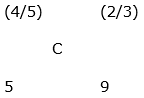[(2/3) – C]: [C – (4/5)] = 5: 9

6 – 9C = 5C – 4

14C = 10

C = 5/7

Ratio of milk to water in mixture C = 5: (7 – 5) = 5: 2

Let amount of milk and water in mixture C is ‘5x’ and ‘2x’ respectively.

Cost price of mixture C = 5x * 1 = 5x

Selling price of mixture C = (5x + 2x) * 0.85 = 5.95x

Profit amount = 5.95x – 5x = 0.95x

Required percent = (0.95x/5) * 100 = 19%

Let initial amount of milk in mixture D is ‘x’.

Amount of milk left in the mixture D = x[1 – 56/280]2 = 134.4

0.64x = 134.4

x = 210

Ratio of milk to water in mixture D = 210: (280 – 210) = 210: 70 = 3: 1

By the rule of alligation: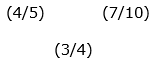Ratio in which mixtures R and T are mixed = [(7/10) – (3/4)]: [(3/4) – (4/5)]

= (1/20): (1/20)

= 1: 1